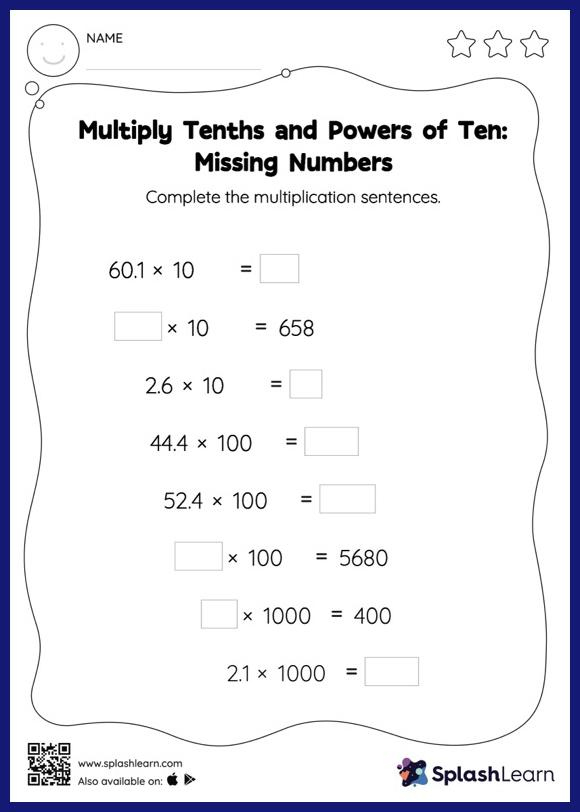# Multiply Tenths and Powers of Ten: Missing Numbers Worksheet

Home > Multiply Tenths and Powers of Ten: Missing NumbersMultiply tenths and powers of ten worksheet helps students develop proficiency with multiplication. Students understand that multiplying a decimal by a power of 10 moves the decimal point to the right by the same amount as the number of zeros in the power of 10. They use this concept to find the missing number in multiply tenths and powers of ten worksheet. In each problem, the numbers are laid out in the horizontal format. Students should try to use different strategies involving composing and decomposing numbers to solve these problems. This will help them develop flexibility and fluency.